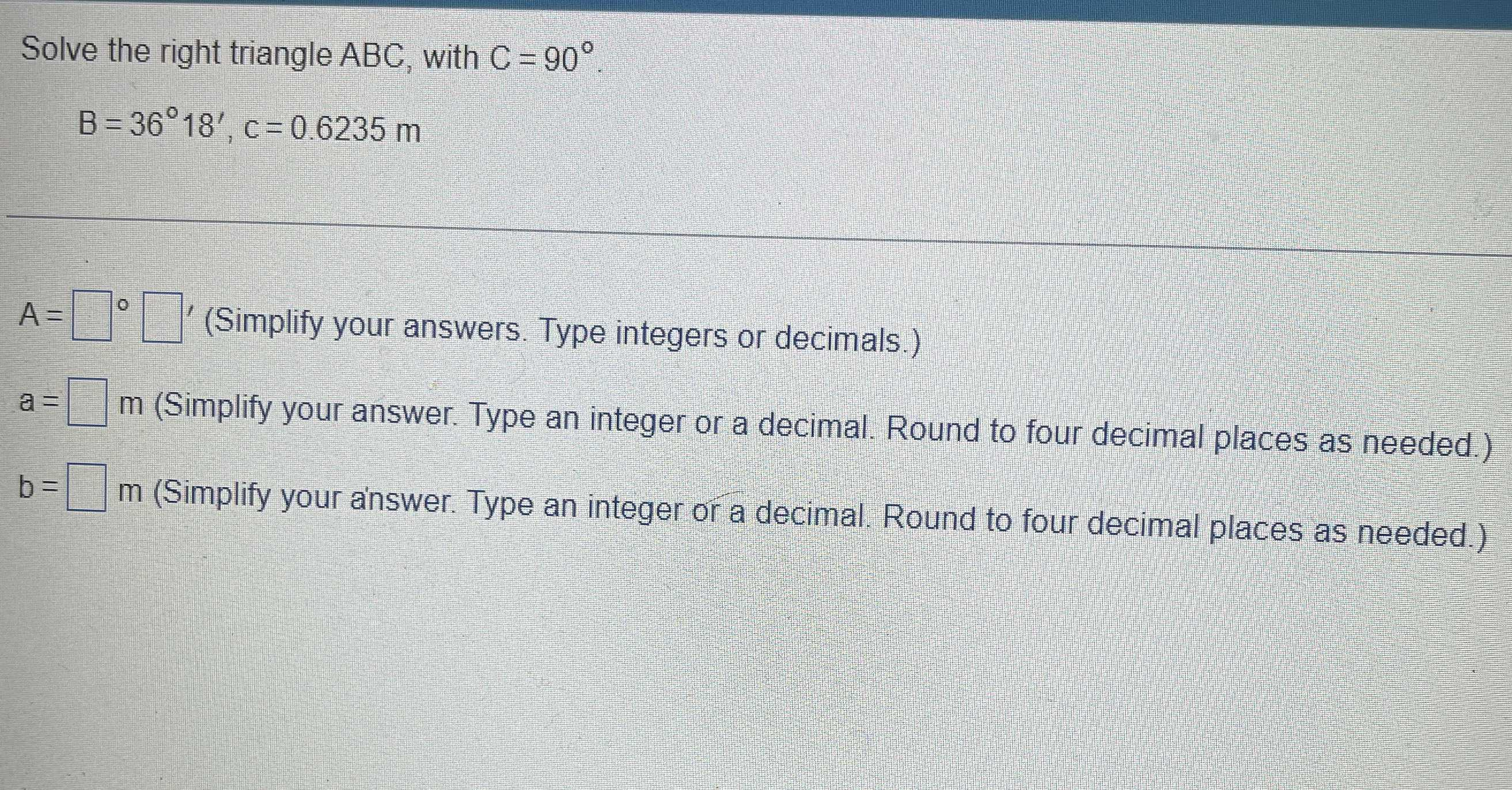### Still have math questions?

Trigonometry
QuestionSolve the right triangle $$A B C$$ , with $$C = 90 ^ { \circ }$$ .

$$B = 36 ^ { \circ } 18 ^ { \prime } , c = 0.6235 m$$

$$A = \square \circ \square$$ ' (Simplify your answers. Type integers or decimals.)

$$a = \square m$$ (Simplify your answer. Type an integer or a decimal. Round to four decimal places as needed.)

$$b = \square m$$ (Simplify your answer. Type an integer or a decimal. Round to four decimal places as needed.)

Side $$a = 0.5025$$
Side $$b = 0.36912$$
Angle $$\angle A = 53.7 ^ { \circ } = 53 ^ { \circ } 42 ^ { \prime } 0 ^ { \prime \prime }$$